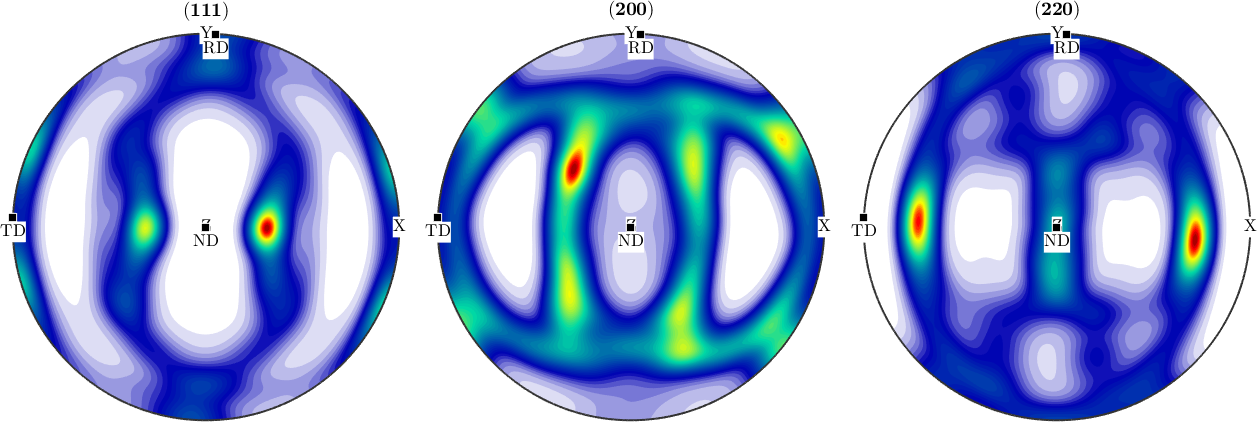Detection of Sample Symmetry edit page

## A synthetic example

We start by modeling a orthotropic ODF with cubic crystal symmetry.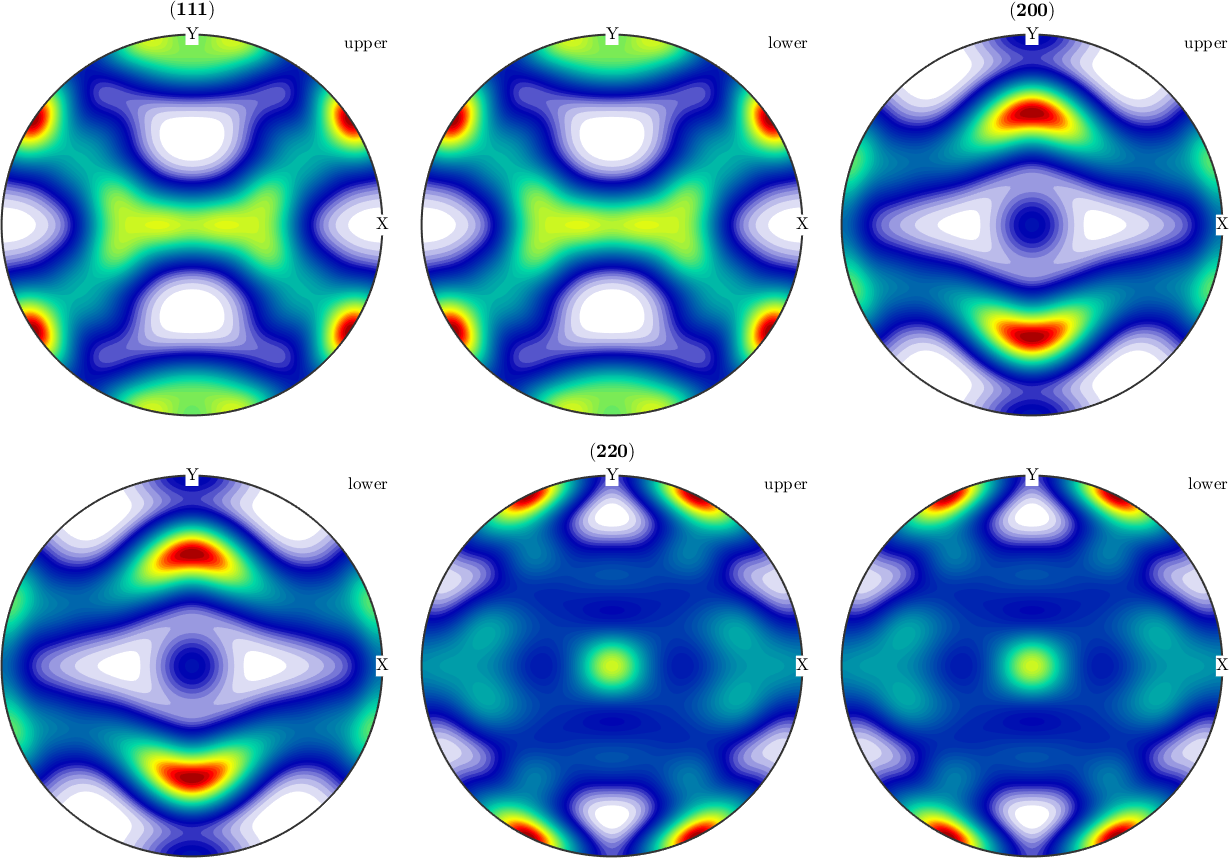## Reconstruct an ODF from simulated EBSD data

Next we simulated some EBSD data, rotate them and estimate an ODF from the individual orientations.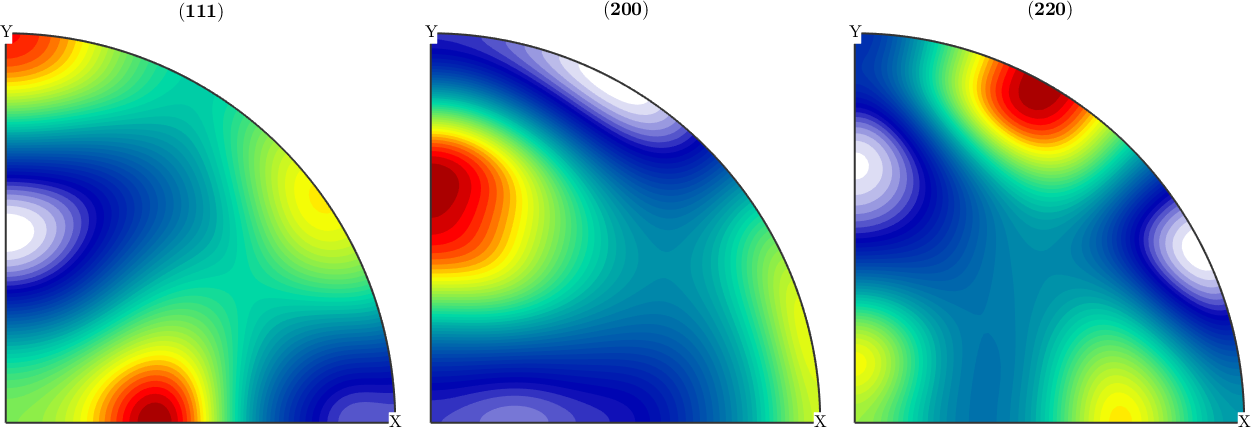## Detect the sample symmetry axis in the reconstructed ODF

We observe that the reconstructed ODF has almost orthotropic symmetry, but with respect to axes different from x, y, z. With the command centerSpecimen we can determine an rotation such that the rotated ODF has almost orthotropic symmetry with respect to x, y, z. The second argument is some starting direction where MTEX looks for a symmetry axis.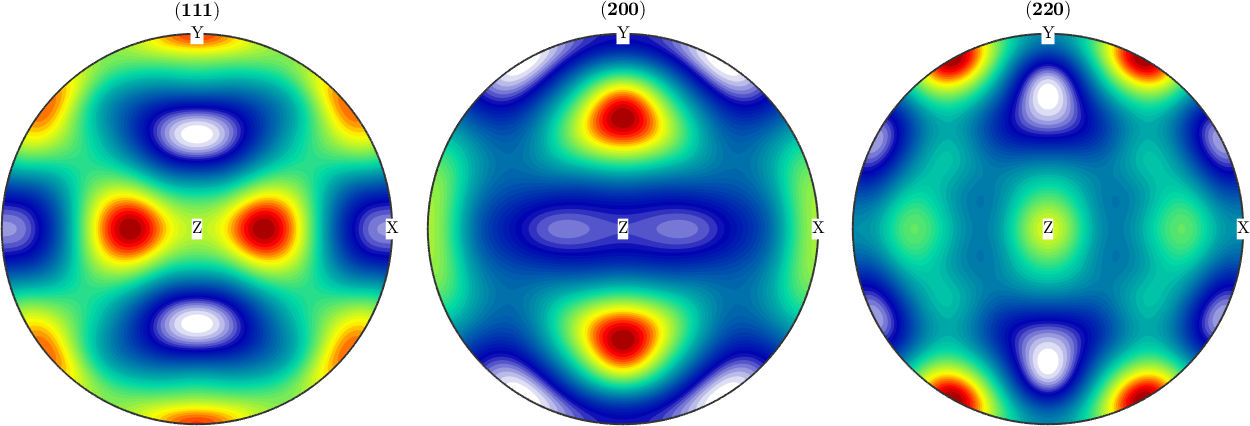## Sample symmetry in an ODF computed from pole figure data

In the next example we apply the function centerSpecimen to an ODF estimated from pole figure data. Lets start by importing them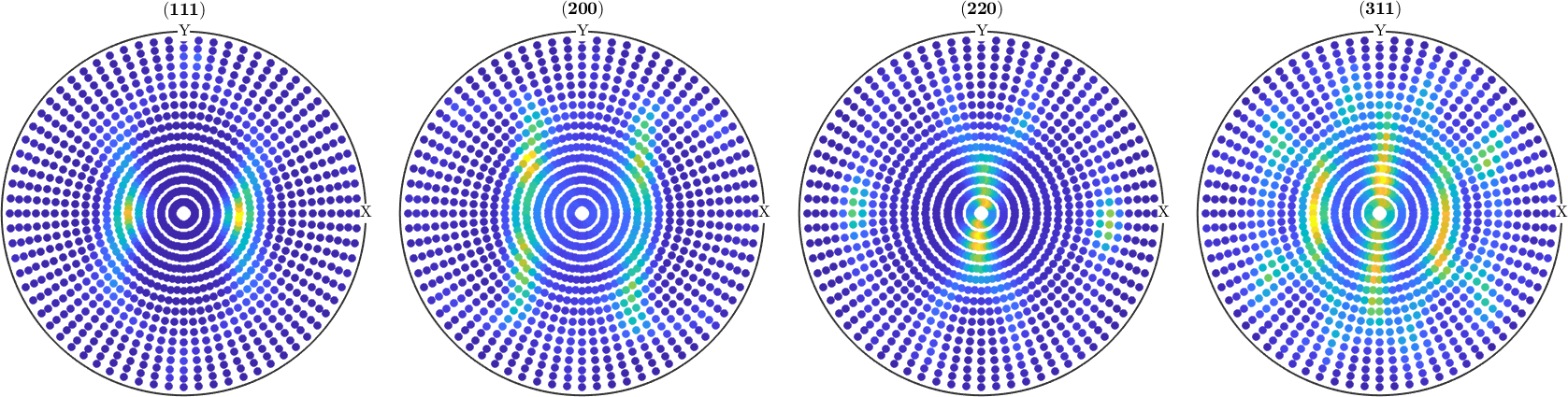In a second step we compute an ODF from the pole figure data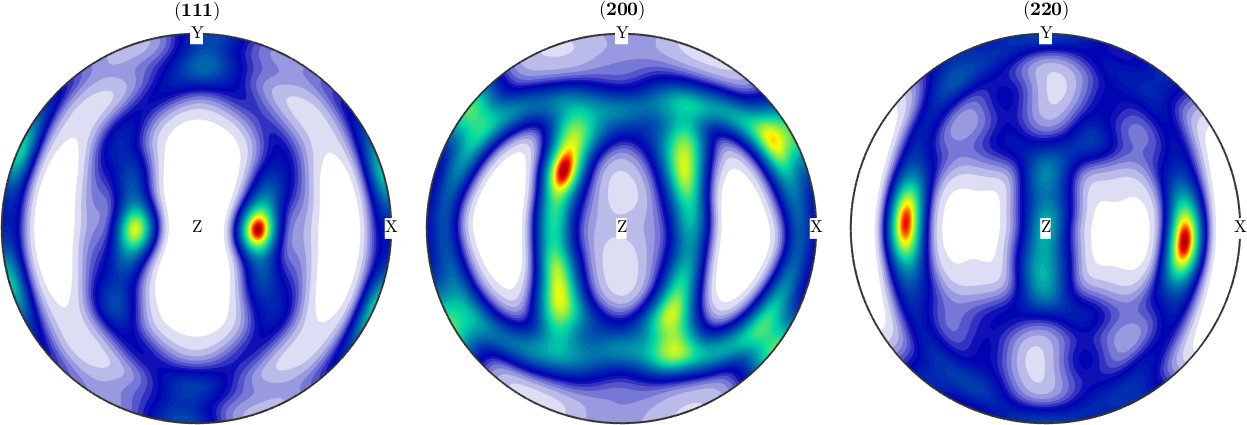Finally, we detect the orthotropic symmetry axes a1, a2, a3 by